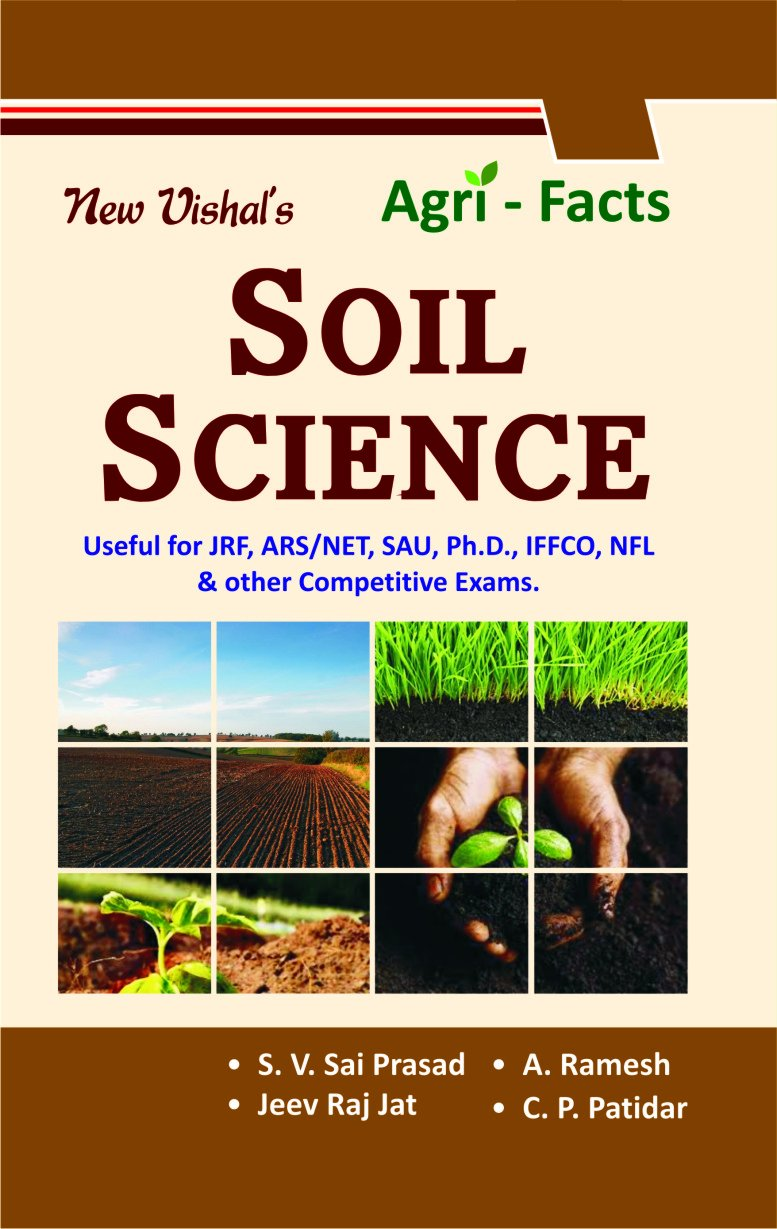# Soil SciencePrice: ₹222.00
(as of Jun 08,2023 12:21:15 UTC – Details)

The book is useful for ICAr’s JRF, ARS/NET, SAUs, Ph.D., IFFCO, NFL and other competitive exams. It includes objective questions on Soil Science, General Agriculture and Important Tables. Contents : A. Soil Science 1. Model Paper – 1 2. Model Paper – 2 3. Model Paper – 3 4. Model Paper – 4 5. Model Paper – 5 6. Model Paper – 6 7. Model Paper – 7 8. Model Paper – 8 9. Model Paper – 9 10. Model Paper – 10 11. Model Paper – 11 12. Model Paper – 12 13. Model Paper – 13 14. Model Paper – 14 15. Model Paper – 15 16. Model Paper – 16 17. Model Paper – 17 18. Model Paper – 18 19. Model Paper – 19 20. Model Paper – 20 B. General Agriculture 1. Model Paper – 1 2. Model Paper – 2 3. Model Paper – 3 4. Model Paper – 4 5. Model Paper – 5 6. Model Paper – 6 7. Model Paper – 7 8. Model Paper – 8 9. Model Paper – 9 10. Model Paper – 10 11. Model Paper – 11 12. Model Paper – 12 C. SHORT NOTES D. Scientists and their contributions E. Glossary of Soil Science

Publisher‏:‎New Vishal Publication; 1st edition (1 January 2016)
Language‏:‎English
Paperback‏:‎456 pages
ISBN-10‏:‎8183990134
ISBN-13‏:‎978-8183990134
Item Weight‏:‎540 g
Dimensions‏:‎22 x 14 x 2.5 cm